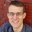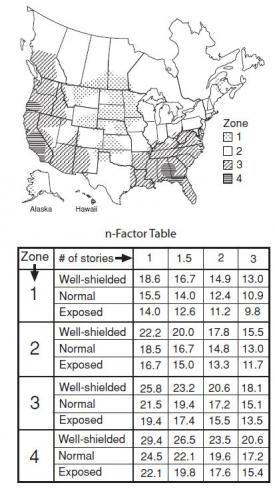Question-and-Answer Resource for the Building Energy Modeling Community
Get s tarted with the Help page

# how to set air infiltration under 50 pa pressureI am doing a passive house model, the required airtightness is less than 0.6 ACH at 50 pa difference. In Opensutdio, in space infiltration design flow rate rates, i can set 0.6 air changes per hour but the question is how to set 50 Pascal pressure difference?

edit retag close merge delete

Sort by » oldest newest most votedYou will need to convert the 0.6ACH50 value to a value at "natural pressure" conditions.

There are several ways to do this, of varying speed and accuracy.

What is typically done in residential calculations is to use a correction factor, known as an "n-factor" or "LBL factor" (after Lawerence Berkeley Lab, where it was developed) to convert your air change rate at 50 pascals (ACH50) to an air change rate at natural pressure (ACHnat).

ACHnat = ACH50/n

The n-factor comes from a series of corrections, based on climate zone (temperature influence), the number of stories (stack effect influence), and how exposed the building is (wind influence). Here is a table for looking up the n-factor for U.S. locations:If you would like a more rigorous approach, you can derive the factor for your application by doing an hourly calculation with local weather data, similar to how the n-factors were determined above, or do a full airflow-network model in CONTAM or related pressure-network software. Consult the ASHRAE Handbook of Fundamentals chapter on Infiltration and Ventilation for equations on residential infiltration (16.24 in ASHRAE HOF 2017).

more

Thanks for your comment! So if the n-factor is 10, then the air infiltration rate is 0.06 ACHnat?

That is correct. If you have multiple spaces in the model, I would suggest calculating the total infiltration rate (cfm or m3/s), then dividing that by the exterior facade area (walls+roof) and assigning that in your model as a flow rate per exterior facade area. That way, infiltration is modeled in spaces proportional to their exterior surface area.

Matt - what is the source for your table of n-Factors above? Thanks!